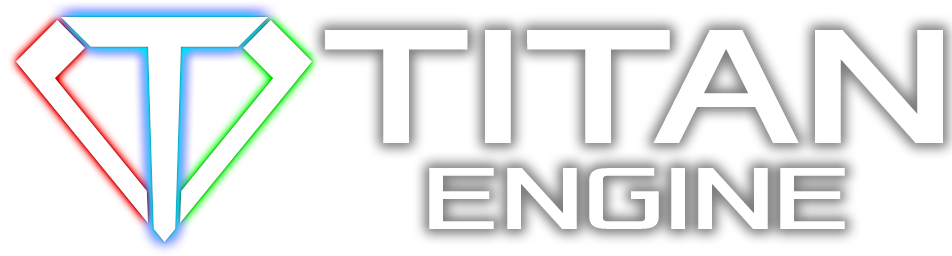About Store Forum Documentation Contactweapon attach Author Message
khcesMember

 Post: #1 weapon attach ^^hi source code see... Code: ```if(slot[SLOT_ARM_R].valid())    {       if(C OrientP *point=owner.cskel.findPoint("HandR"))       {          Item   &item  =slot[SLOT_ARM_R]();          Matrix  matrix=item.matrix(); // get current matrix          item.matrix(Matrix().setPosDir(point->pos,point->cross(),point->dir)); // set new matrix          item.update();          // immediately overwrite item members after calling 'update'          Vec vel,ang_vel; GetVel(vel,ang_vel,matrix,item.matrix()); // calculate velocities between old and new matrixes          item.setDrawingVelocities(vel,ang_vel); // put the velocities to be used in motion blur drawing       }    }``` Look at the code... using OrientP CSkelBone as possible ? Not OrientP Max makes the bone can attach weapons My english sorry thanks ^^
04-06-2011 05:44 PM
EsenthelPost: #2 RE: weapon attach Hi, yes you can use skeleton bone just transform i-th Skeleton::bones by CSkeleton::bone(i).matrix() it will give you skeleton bone matrix, animated, in world space
04-06-2011 05:48 PM
khcesMember

 Post: #3 RE: weapon attach hi, Fast Answer thanks ^^ if(slot[SLOT_ARM_R].valid()) { if(C OrientP *point=owner.cskel.findPoint("HandR")) { Item &item =slot[SLOT_ARM_R](); Matrix matrix=item.matrix(); // get current matrix CSkelBone* sword=owner.cskel.findBone("Sword"); Matrix mat_dummy=sword->matrix(); < --- this use? // item.matrix(Matrix().setPosDir(point->pos,point->cross(),point->dir)); // set new matrix item.update(); // immediately overwrite item members after calling 'update' Vec vel,ang_vel; GetVel(vel,ang_vel,matrix,item.matrix()); // calculate velocities between old and new matrixes item.setDrawingVelocities(vel,ang_vel); // put the velocities to be used in motion blur drawing } } (This post was last modified: 04-06-2011 06:46 PM by khces.)
04-06-2011 06:45 PM
EsenthelSadaharPost: #5 RE: weapon attach Im interested in this issue... I dont get what to do to get the weapon correctly displayed :/ Currently I have that, the weapon is correctly positioned but (the club for example) is vertical, so I guess the orientation parts arent correct or even considered in the matrixes :( EDIT: OK! I just read carefull (4:37 am, cant sleep ^^) and got it workingHere is the code if someone needs it: Code: ```if(slot[SLOT_ARM_R].valid())    {       if(CSkelBone* bone=owner.cskel.findBone("Weapon_R_Bone"))       {          Item   &item  =slot[SLOT_ARM_R]();          Matrix  matrix=item.matrix(); // get current matrix          OrientP point = *owner.cskel.skeleton()->findBone("Weapon_R_Bone");          point*= bone->matrix();          item.matrix(Matrix().setPosDir(point.pos,point.cross(),point.dir)); // set new matrix          Vec vel,ang_vel; GetVel(vel,ang_vel,matrix,item.matrix()); // calculate velocities between old and new matrixes          item.setDrawingVelocities(vel,ang_vel); // put the velocities to be used in motion blur drawing       }    }``` (This post was last modified: 07-30-2011 03:38 AM by Sadahar.)